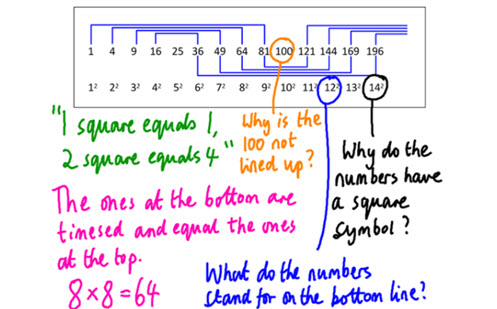Go to website

# Index patterns

Students begin this lesson sequence by discussing the number line, ensuring students understand its use. Then students are introduced to exponents both negative and postive, observing how this can alter an answer. Students work collaboratively identifying patterns when changing the power and the base. Students then move on to cube numbers completing similar tasks.

Year level(s) Year 8
Audience Teacher
Purpose Content knowledge, Student task, Teaching resource, Teaching strategies, Planning support
Teaching strategies and pedagogical approaches Collaborative learning, Differentiated teaching, Explicit teaching, Feedback, Questioning, Setting goals, Structuring lessons, Worked examples
Keywords powers, base, cube, integers, indices, negative, postive, exponent, squares

## Curriculum alignment

Curriculum connections ICT general capability, Numeracy
Strand and focus Number, Build understanding, Apply understanding
Topics Indices, Operating with number, Patterns and algebra, Addition and subtraction, Multiplication and division, Place value
AC: Mathematics (V9.0) content descriptions
AC9M8N02
Establish and apply the exponent laws with positive integer exponents and the zero-exponent, using exponent notation with numbers